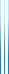# Courses

236315 Algebraic Methods Spring 2017

A course involving various topics in TCS with an algebraic flavor.

Here is the complete syllabus:

1. The extended Euclidean algorithm, exponentiation by repeated squaring, a polynomial algorithm for SAT.
2. Different computation models (transdichotomous, bit complexity, arithmetic complexity), Karatsuba’s integer multiplication algorithm.
3. Strassen’s matrix multiplication algorithm, tensors and tensor rank.
4. Tensor rank and the exponent of matrix multiplication.
5. Tensor rotation, tensor product, direct sum of tensors, border rank.
6. Asymptotic sum inequality, the Coppersmith–Winograd algorithm (briefly).
7. Discrete Fourier transform.
8. Fast Fourier transform.
9. Schönhage–Strassen integer multiplication algorithm.
10. Primality tests: Fermat, Solovay–Strassen, Miller–Rabin (without proof), AKS (only briefly mentioned).
11. Integer factorization algorithms: Pollard’s rho, Pollard’s $p-1$, the elliptic curve method.
12. Guest talk by the teaching assistant (Tamer Mour) on factorization using elliptic curves.
13. More integer factorization algorithms: quadratic sieve, number field sieve (briefly).

I prepared lecture notes for some of these subjects:

More details are available on webcourse.